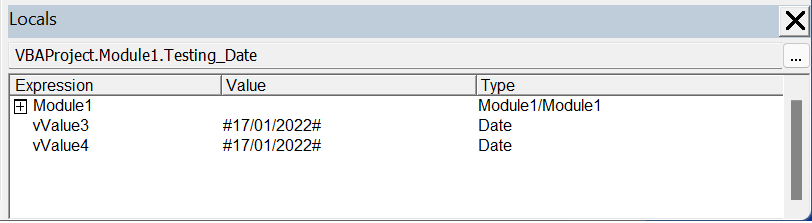# Date Data Type

A Date data type can hold any date from 1 Jan 0100 0:00:00 to 31 Dec 9999 23:59:59.
Using the Date data type is the best way to work with dates (and times) in VBA.
Variables that are declared as a Date data type are actually stored as a decimal number.
This data type uses 8 bytes
The default value is (?) 00:00:00
This data type can be used to store dates and times

`Dim dtMyDate As Date `

`Dim vValue3 As Date Dim vValue4 As Date vValue3 = CDate(VBA.Date) vValue4 = VBA.Date() `### Excel

It is important to remember that the range of a date in VBA is greater than the range accepted by Excel.
Dates in Excel only start at 1 Jan 1900.
The integer portion represents the number of days since December 30, 1899. Any dates before this one are stored as negative numbers; all dates after are stored as positive values. If you convert a date value representing December 30, 1899, to a double, you'll find that this date is represented by zero.
The decimal portion of a date represents the amount of time that has passed since midnight. For example, if the decimal portion of a date value is .75, three-quarters of the day has passed, and the time is now 6 P.M.
Because the integer portion of a date represents number of days, you can add and subtract days from one date to get another date.

CLng(dtMyDate)